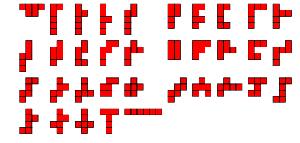## Tuesday, June 9, 2009

### 35

35 = 5 x 7.

35 is the sum of the cubes of the first two primes: 23 + 33 = 35.

The product of
35 and 36 has the same prime factors as the product of 4374 and 4375.

35 can be written as a sum of odd primes in 35 ways, and as a sum of primes in 35 x 5 ways.

35 has a unique representation as a sum of three squares: 35 = 12 + 32 + 52.

35 is the smallest number with five representations as a sum of three distinct primes: 35 = 3 + 13 + 19 = 5 + 7 + 23 = 5 + 11 + 19 = 5 + 13 + 17 = 7 + 11 + 17.

35 is the fifth tetrahedral number (the sum of the first five triangular numbers).

35 is the fourth pentatope number.

35 is 55 in base 6.There are
35 hexominoes, polyominoes made from six squares.Source:
Number Gossip